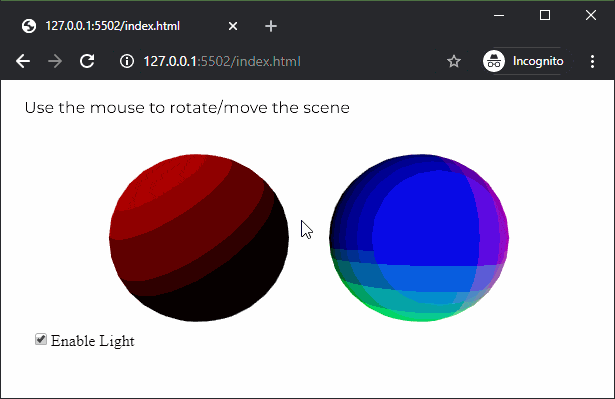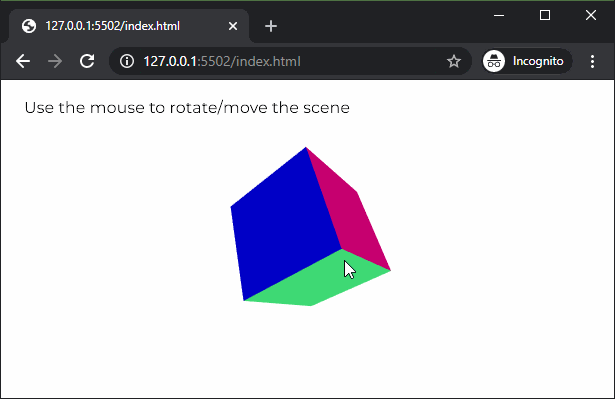GeeksforGeeks App
Open AppBrowser
Continue

# p5.js | normalMaterial() Function

The normalMaterial() function in p5.js is used to create a normal material for an object. A normal material is not affected by any light and also does not reflect any light. The surface facing the x-axis becomes red, the surface facing the y-axis becomes green and the surface facing the z-axis becomes blue. It is usually used as a placeholder material for debugging.

Syntax:

`normalMaterial()`

Parameters: This function does not accept any parameters.

Below example illustrates the normalMaterial() function in p5.js:

Example 1: This example shows that the normal material is not affected by light.

 `let newFont;``let hasLight = ``true``;`` ` `function` `preload() {``  ``newFont = loadFont(``'fonts/Montserrat.otf'``);``}`` ` `function` `setup() {``  ``createCanvas(600, 300, WEBGL);``  ``textFont(newFont, 16);`` ` `  ``lightCheckbox = createCheckbox(``'Enable Light'``, ``true``);``  ``lightCheckbox.position(30, 250);``  ``lightCheckbox.changed(() => hasLight = !hasLight);``}`` ` `function` `draw() {``  ``background(``'white'``);``  ``fill(``'black'``);`` ` `  ``text(``"Use the mouse to rotate/move the scene"``, -285, -125);``  ``noStroke();``  ``orbitControl();`` ` `  ``// Enable lights when the checkbox is checked``  ``if` `(hasLight)``    ``directionalLight(color(``'red'``), height / 2, width / 2, -250);`` ` `  ``// Draw the sphere which uses ambient material``  ``ambientMaterial(255);``  ``translate(-100, 0, 0);``  ``sphere(80);``  ``translate(100, 0, 0);`` ` `  ``// Draw sphere which uses normal material``  ``normalMaterial();``  ``translate(100, 0, 0);``  ``sphere(80);``  ``translate(-100, 0, 0);``}`

Output:Example 2: This example shows the colors for the different axes of the normal material.

 `let newFont;``let hasNormalMaterial = ``true``;`` ` `function` `preload() {``  ``newFont = loadFont(``'fonts/Montserrat.otf'``);``}`` ` `function` `setup() {``  ``createCanvas(600, 300, WEBGL);``  ``textFont(newFont, 16);``}`` ` `function` `draw() {``  ``background(``'white'``);``  ``fill(``'black'``);`` ` `  ``text(``"Use the mouse to rotate/move the scene"``, -285, -125);``  ``// text("Select directional light color", -285, -100);``  ``shininess(10);``  ``noStroke();``  ``orbitControl();`` ` `  ``// Use the normal material``  ``normalMaterial();`` ` `  ``// Create the box``  ``rotateX(60)``  ``rotateY(120)``  ``rotateZ(60)``  ``box(100);``}`

Output:Online editor: https://editor.p5js.org/

My Personal Notes arrow_drop_up
Related Tutorials